Tim Regan

•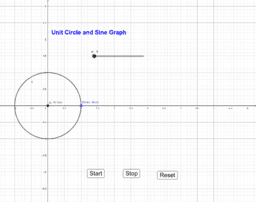Unit Circle and Sine Graph

Activity

Tim Regan

•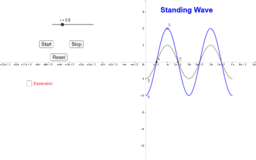Standing Wave

Activity

Tim Regan

•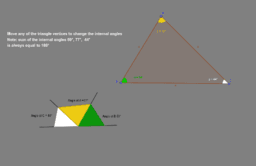Angles in a triangle sum to 180 degrees

Activity

Tim Regan

•Moon phases

Activity

Tim Regan

•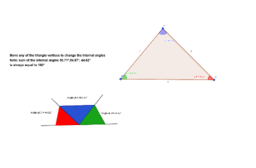Angles in any triangle always sum to 180°

Activity

Tim Regan

•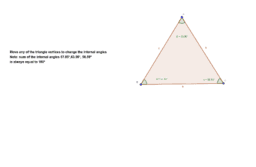Internal angles in any triangle sum to 180°

Activity

Tim Regan

•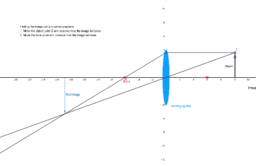Converging lens

Activity

Tim Regan

•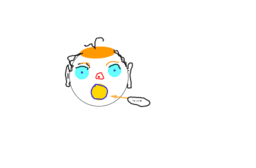Irish face

Activity

Tim Regan

•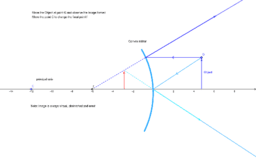Convex mirror ray diagram

Activity

Tim Regan

•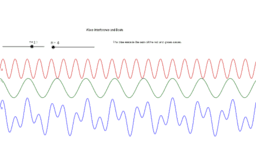Travelling wave interference

Activity

Tim Regan

•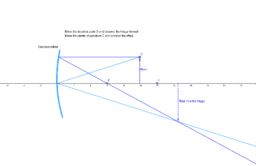Concave mirror images

Activity

Tim Regan

•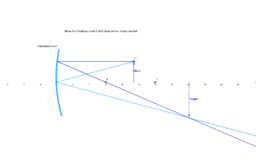Concave Mirror

Activity

Tim Regan

•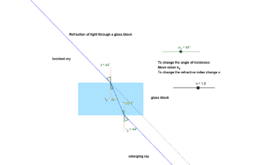Refraction in Glass

Activity

Tim Regan

•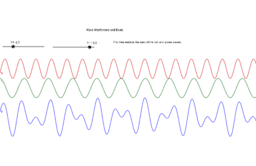Wave Interference

Activity

Tim Regan

•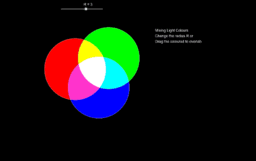Mixing Light Colours

Activity

Tim Regan

•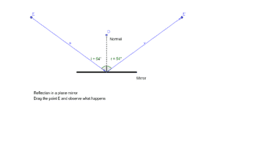Activity

Tim Regan